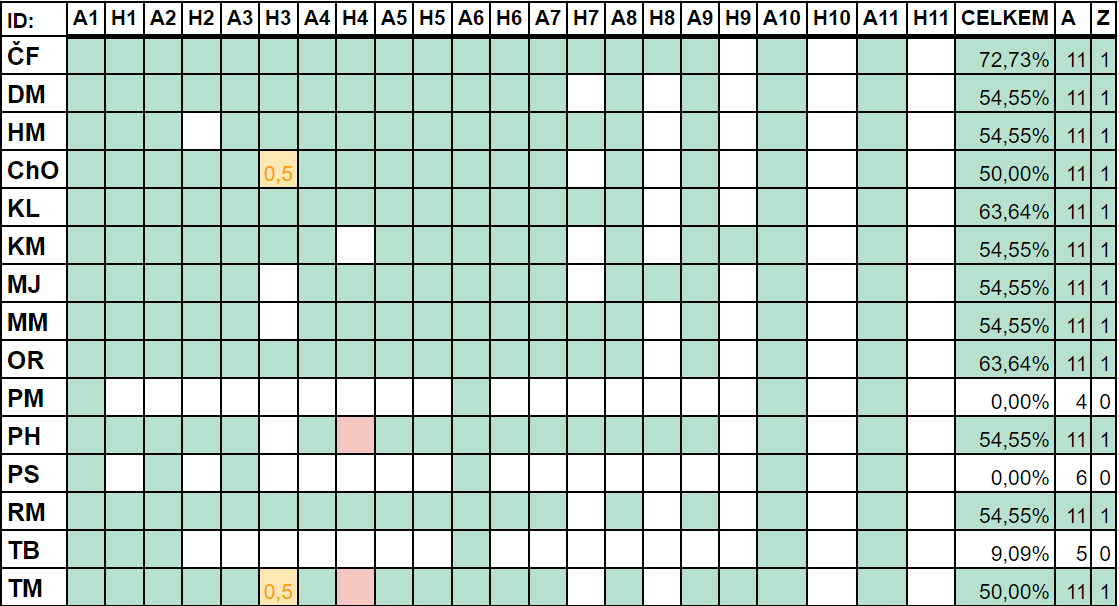# Applications of LA in Combinatorics

Tutorials for Applications of Linear Algebra in Combinatorics [NDMI028] in winter semester 2022/2023. Tutorials take place on Friday 15:40 at K12, Karlín. I lead the tutorials together with prof. Kratochvíl.

## Course completion requirements

It is required to attend all tutorials and submit solution to home assignments in order to pass the course tutorials. There are two types of homework:

1. *-type: Every week, one assignment will be posted and you have two weeks to finish it. To pass the tutorials, you have to submit at least half of the assignments.
2. a-type: If you do not attend tutorials, you have to submit homework assignments corresponding to the tutorials.

## HW submission progress## Homework assignments

7. 10. 2022 Revision of Linear Algebra here 21. 10. 2022
14. 10. 2022 Determinants and Odd/Even cities here 28. 10. 2022
21. 10. 2022 Odd cities here 4. 11. 2022
28. 10. 2022 National holiday here 28. 10. 2022
4. 11. 2022 Mod-q cities here 18. 11. 2022
11. 11. 2022 Spectral graph theory I. here 25. 11. 2022
18. 11. 2022 Spectral graph theory II. here 2. 12. 2022
25. 11. 2022 Spectral graph theory III. here 16. 12. 2022
2. 12. 2022 Spectral graph theory IV. here 16. 12. 2022
6. 12. 2022 Spectral graph theory V. here 6. 1. 2023

## Lectures

The lecture notes are avalaible only in Czech. For materials in English, contact prof. Kratochvil at honza@kam.mff.cuni.cz.

Date: Topic: Materials:
7. 10. 2022 Matrix representations of graphs, powers of the adjacency matrix, determinants and spanning trees here
14. 10. 2022 Linear (in)dependece, sizes of special set systems, 2-distances sets here
21. 10. 2022 Orthogonality and orthogonal complements, cycle space of a graph here
28. 10. 2022 National holiday here
4. 11. 2022 Linear forms, dual homomorphisms, Seidel switching here
11. 11. 2022 Spectral graph theory, eigenvalues of graphs, Moore graphs here
18. 11. 2022 Strongly regular graphs, friendship theorem, interlacing of eigenvalues here
25. 11. 2022 Applications of interlacing of eigenvalues here
2. 12. 2022 Shannon capacity of graphs here
9. 12. 2022 Coding theory, Lloyd theorem here